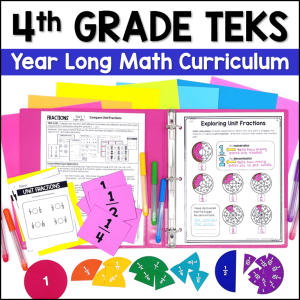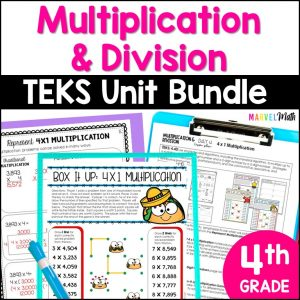Save 10% on your first order with code EXTRA10

# 2×2 and 3×1 Multiplication Game: Partial Products, Area Models, Arrays & More

\$4.00187.25\$30.99
SKU: 3794397 Category:

## Description

This resource will give your students fun & meaningful practice with partial products, area models, arrays, pictorial models and the commutative and distributive properties. Students will make connections between the multiplication representations for 2×2 and 3×1 problems while engaging in the 6 activities and included competitive game.

Classroom Uses:

??Guided Math Stations

??Tutoring

??Small Group Lessons

??Review

??Test Prep

What’s Included?

?6 Activities

?Fun Game

?6 Emoji Cards & 28 Representation Cards to be used with the Activities and Game

?Key

Color and Black-and-White Versions are Included.

??TEKS Alignment??

Supporting Standards

4.4(C)

represent the product of 2 two-digit numbers using arrays, area models, or equations, including perfect squares through 15 by 15

4.4(D)

use strategies and algorithms, including the standard algorithm, to multiply up to a four-digit number by a one-digit number and to multiply a two-digit number by a two-digit number. Strategies may include mental math, partial products, and the commutative, associative, and distributive properties.

??Common Core Alignment??

4.NBT.B.5

Multiply a whole number of up to four digits by a one-digit whole number, and multiply two two-digit numbers, using strategies based on place value and the properties of operations. Illustrate and explain the calculation by using equations, rectangular arrays, and/or area models.